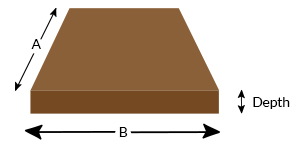## How Much Topsoil Do You Need?

Customers frequently ask us how much topsoil they'll need for their project...

Topsoil is often sold by the metric tonne (1000kg), but the easiest way to calculate the quantity of topsoil you will need is by first calculating the volume (cubic metres or litres).

To save you the trouble of doing the maths, we've created a Topsoil Calculator which will work out how much you'll require.

The calculator should be used as a rough guide to calculate how much topsoil you'll need, but if you are in any doubt, please Contact Us with your measurements and we'll be happy to help.A = B = Units

Depth = Units

Total = Tonnes of Topsoil We Recommend